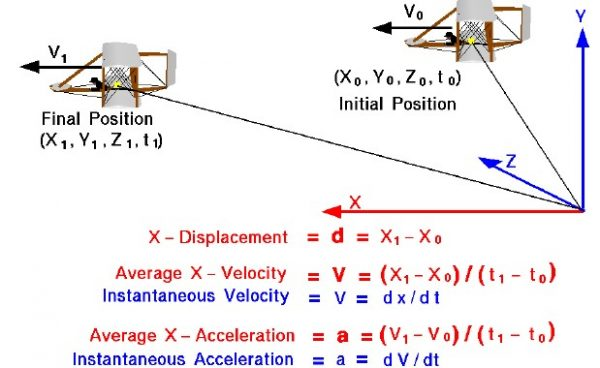# TranslationsWe live in world that is defined by three spatial dimensions and one time dimension. Objects can move within this domain in two ways. An object can translate, or change location, from one point to another. And an object can rotate, or change its attitude. In general, the motion of an aircraft involves both translation and rotation. This makes the analysis of aircraft motion much more complex than ordinary objects.

On this page we will consider only the translation of an object within our domain. We can specify the location of our aircraft at any time (t) by specifying three coordinates (x, y, and z) on an orthogonal coordinate system. An orthogonal coordinate system has each of its coordinate directions perpendicular to all other coordinate directions. Initially, our aircraft is at point “0”, with coordinates x0, y0, and z0 at time t0. In general, the aircraft can move through the domain until at some later time t1 the aircraft is at point “1” with coordinates x1, y1, and z1. We can specify the displacement (d) in each coordinate direction by the difference in coordinate from point “0” to point “1”. The x-displacement equals (x1 – x0), the y-displacement equals (y1 – y0), and the z-displacement equals (z1 – z0). For simplicity on the slide we show only the x coordinate.

### d = x1 – x0

The average velocity (V) of the aircraft through the domain is the displacement divided by the time difference. In the X – direction, the average velocity is (x1 – x0) / (t1 – t0). This is only an average velocity; the aircraft could speed up and slow down inside the domain. At any instant, the aircraft could have a velocity that is different than the average. If we shrink the time difference down to a very small (differential) size, we can define the instantaneous velocity to be the differential change in position divided by the differential change in time (V = dx / dt) from calculus. So when we initially specified the location of our aircraft with (x0, y0, z0, and t0) coordinates, we could also specify an initial V0 instantaneous velocity. Likewise at the final position (x1, y1, z1, and t1), the velocity could change to some V1. (We are here considering only the x-component of the velocity. In reality, the aircraft velocity can change in all three directions. Velocity is a vector quantity; it has both a magnitude and a direction associated with it.)

### V = (x1 – x0) / (t1 – t0)

The average acceleration (a) of the aircraft through the domain is the difference in the velocity divided by the time difference. In the X – direction, the average acceleration is (V1 – V0) / (t1 – t0). As with the velocity, this is only an average acceleration. At any instant, the aircraft could have an acceleration that is different than the average. If we shrink the time difference down to a very small (differential) size, we can define the instantaneous acceleration to be the differential change in velocity divided by the differential change in time (a = dV / dt) from calculus. From Newton’s second law of motion, we know that forces on an object produce accelerations. If we can determine the forces on an aircraft, and how they change, we can use the equations presented on this slide to determine the location and translation of the aircraft.

Provide feedback